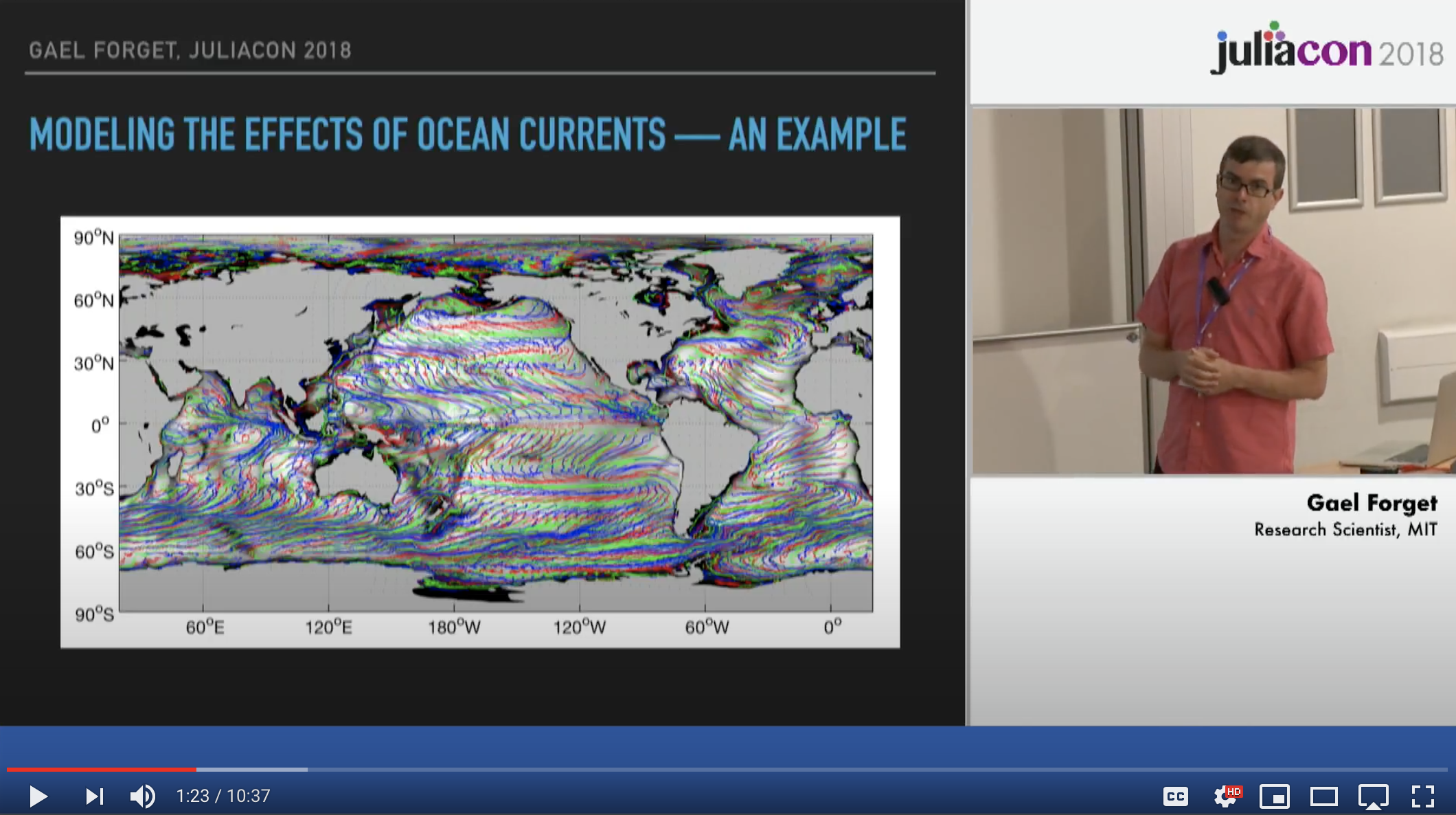# MeshArrays.jl

MeshArrays.jl defines an array type that can contain / organize / distribute collections of inter-connected arrays as done in climate models (see below).

As illustrated in the Global Ocean Notebooks, MeshArrays' data structures can be used to accurately analyze ocean heat transport, material displacements, and many other important topics in climate science.

Contents:

## Installation

using Pkg
Pkg.test("MeshArrays")

Julia's package manager, Pkg.jl, is documented the main Julia doc and here in details.

## Basic Examples

Examples below (1) generate a grid configuration, (2) seed a 2D field of random noise, (3) smooth out this field, and (4) plot subdomain arrays. Smoothing is done via a lateral diffusion equation through time to illustrate how MeshArray computes partial derivatives & transfers data between neighboring subdomains. Examples 2 & 3 illustrate grid configurations commonly used in global models.

[A] 16 subdomains, with 40x40 grid points each, covering a doubly periodic domain

using MeshArrays; p=dirname(pathof(MeshArrays))
γ,Γ=GridOfOnes("PeriodicDomain",16,20)

include(joinpath(p,"../examples/Demos.jl"))
(xi,xo,_,_)=demo2(Γ);
show(xo)

using Plots; plotlyjs()
include(joinpath(p,"../examples/Plots.jl"))
heatmap(xo,clims=(-0.25,0.25))
Grid scale noiseSmoothed noise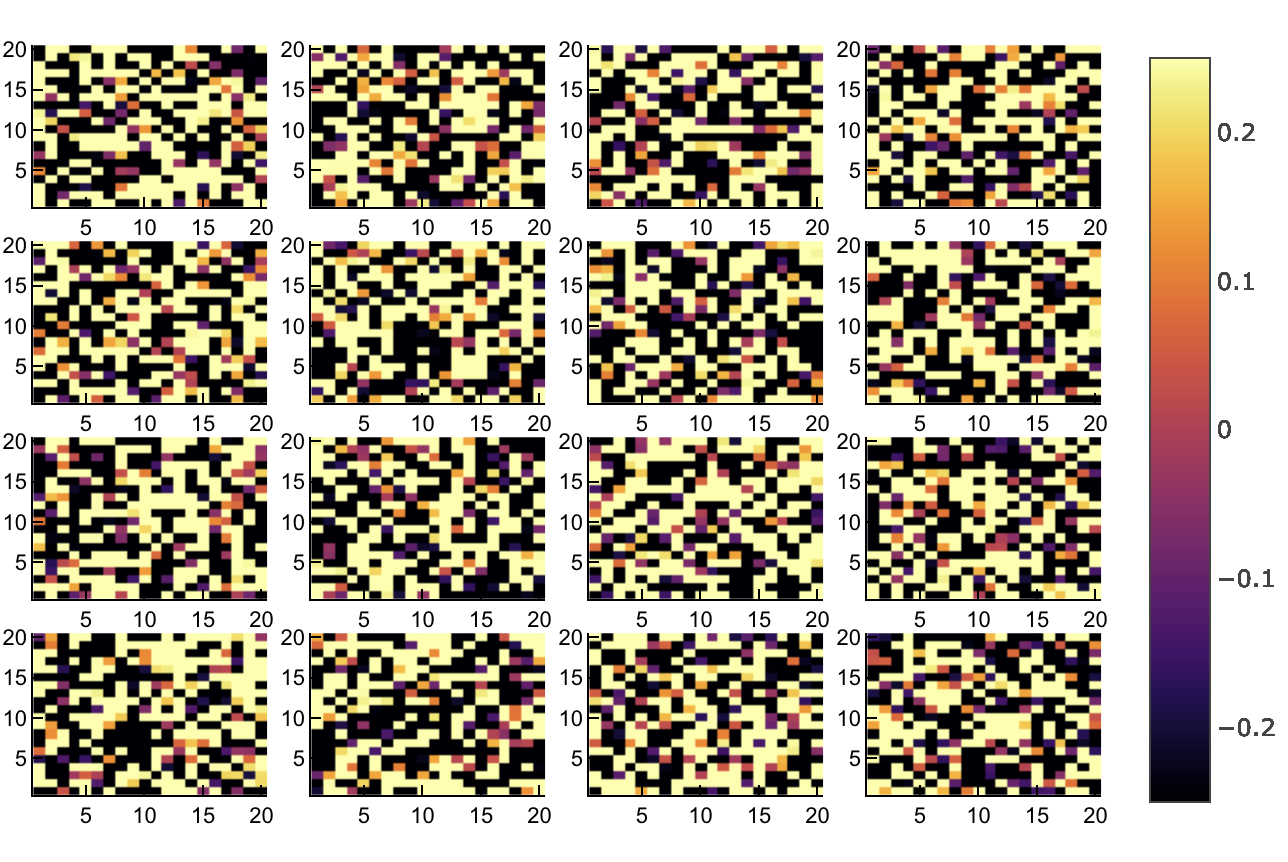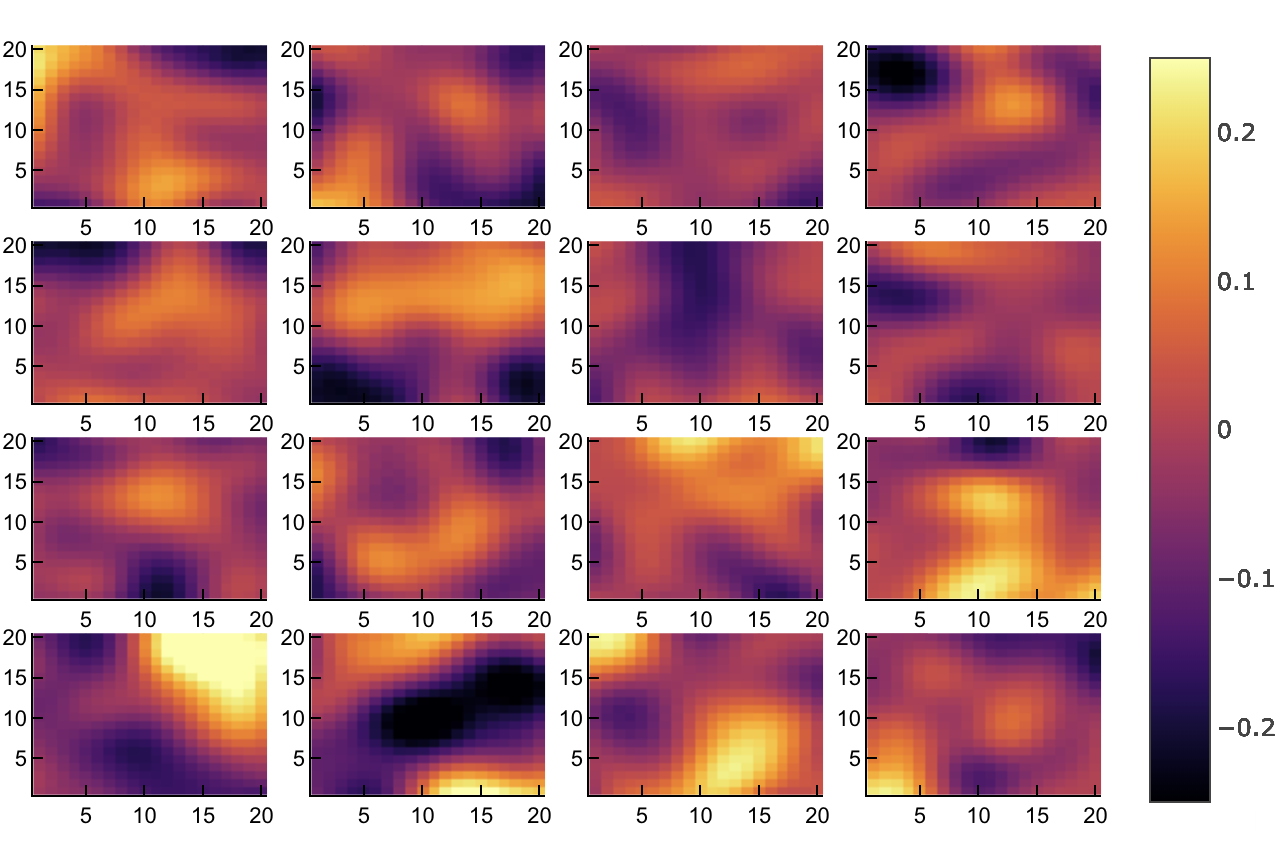[B] 6 subdomains, with 100x100 points each, covering the six faces of a cube

γ,Γ=GridOfOnes("CubeSphere",6,100)
D=demo2(Γ)

[C] Global Model Grid with 5 uneven subdomains, variable spacing, & continents

#run(git clone https://github.com/gaelforget/GRID_LLC90)
D=demo2(Γ)
heatmap(D,clims=(-0.25,0.25))

## Earth Model Grids

-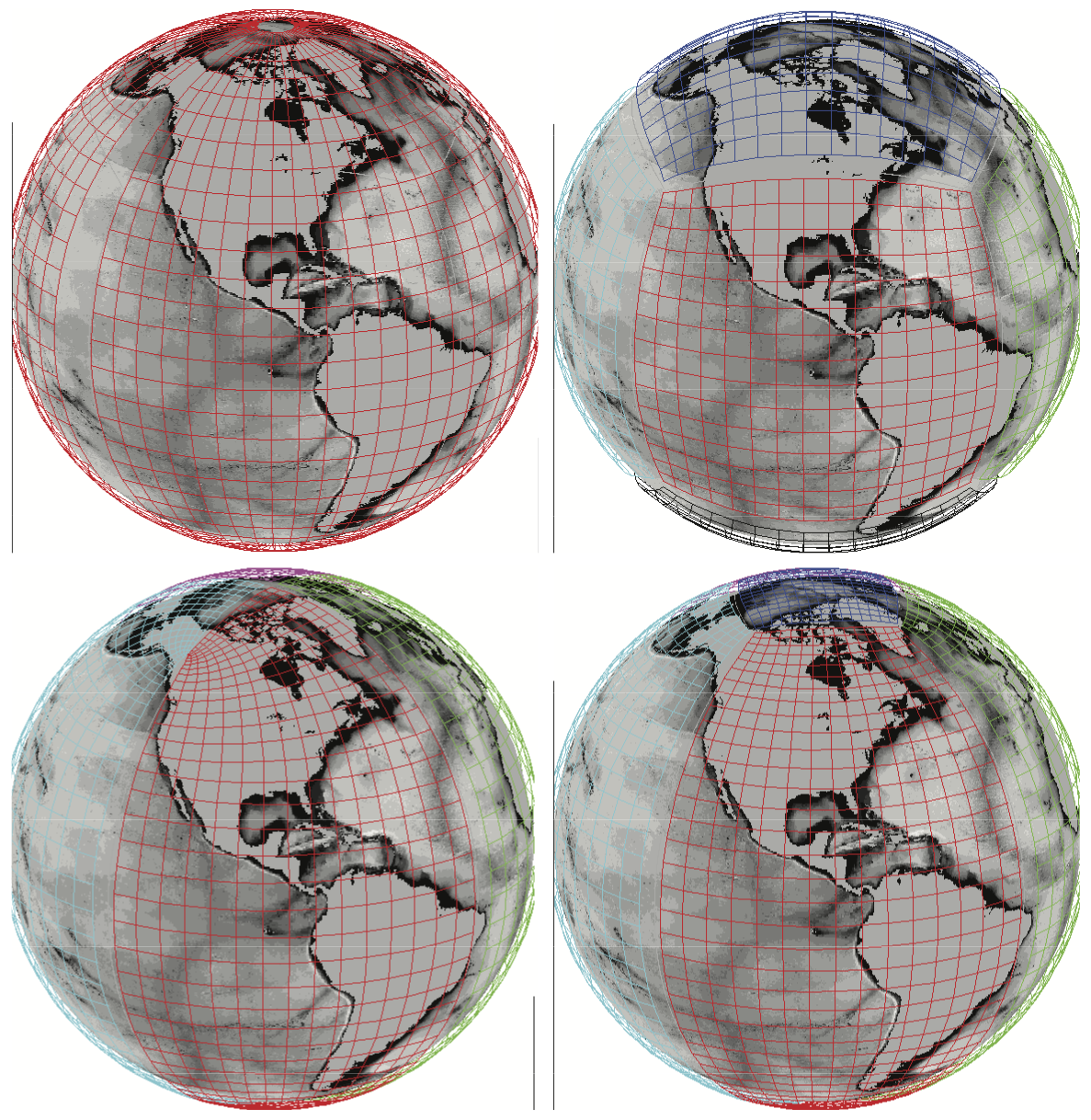## Simulated Ocean Particles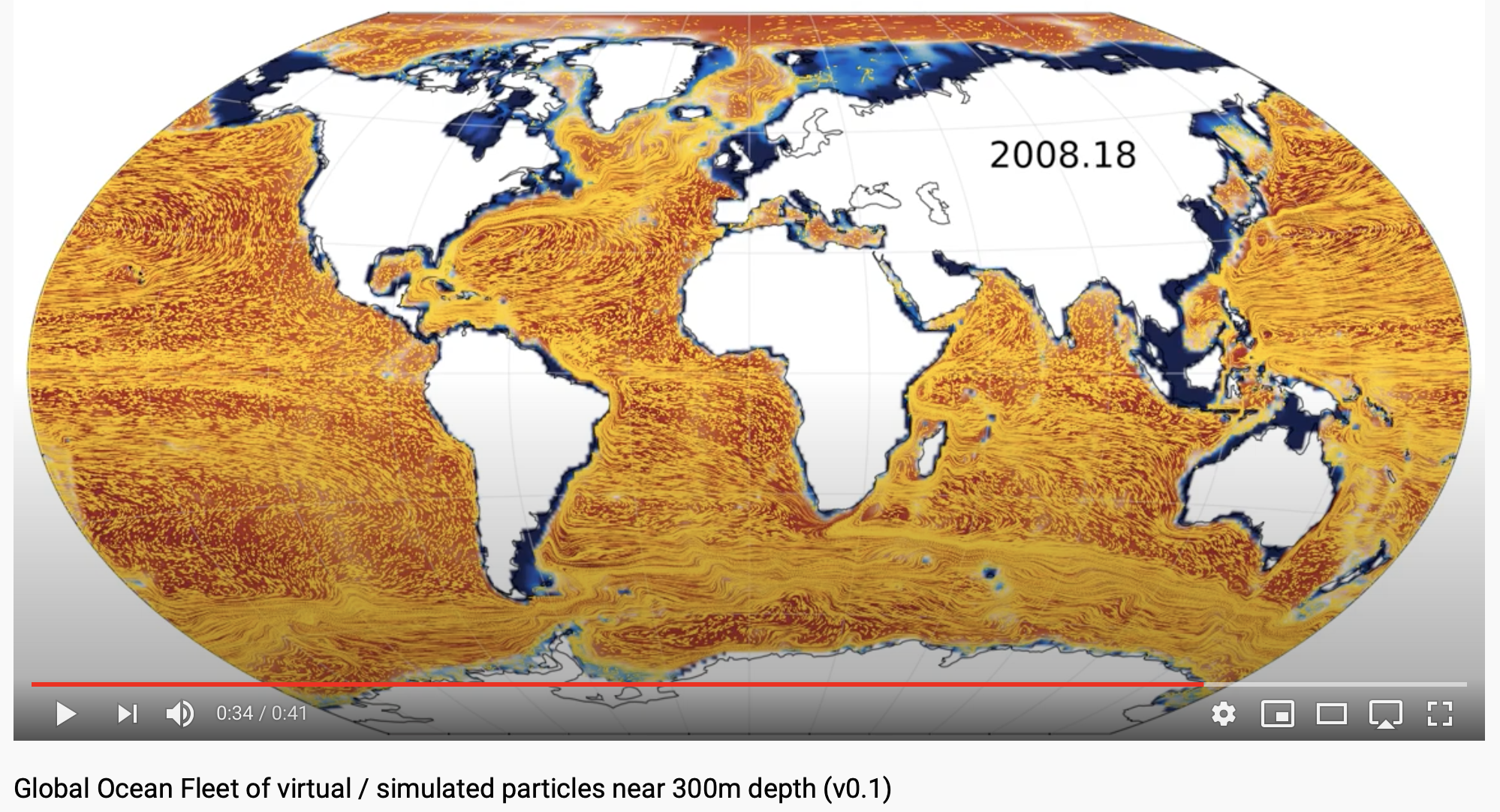## JuliaCon 2018 Video

(where MeshArrays.jl was first introduced as as gcmfaces.jl)